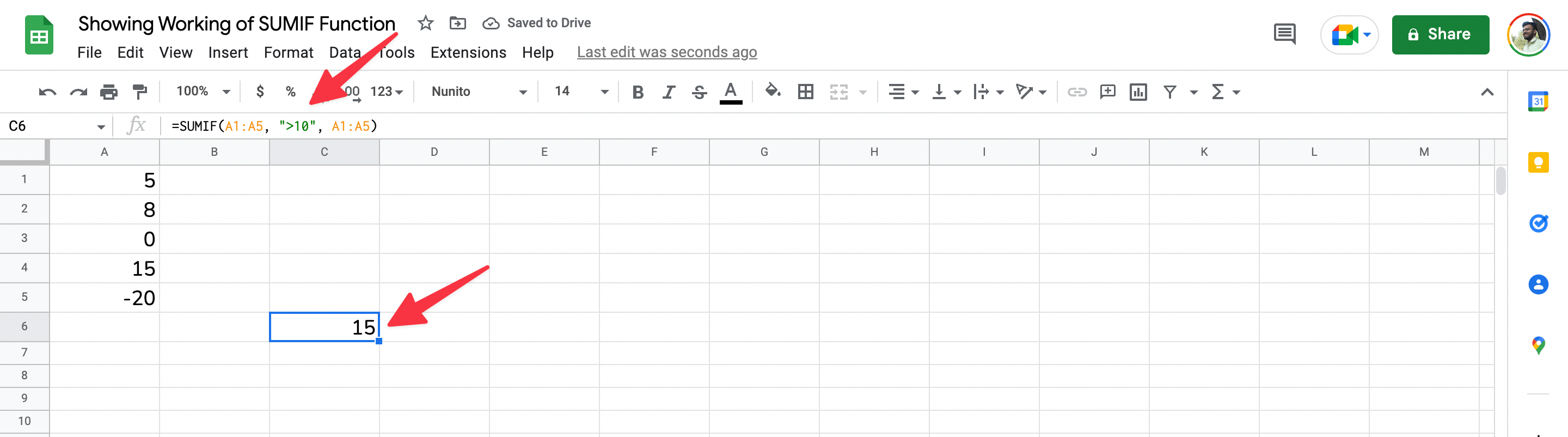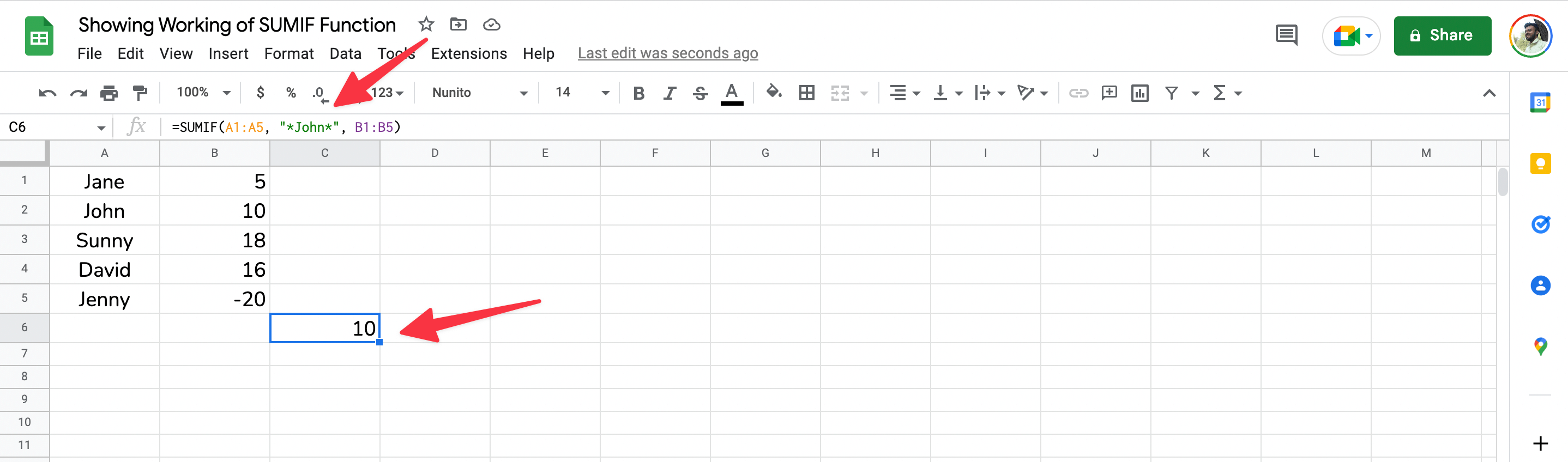# How to Use =SUMIF Function in Microsoft Excel & Google Sheets

The =SUMIF function is a powerful tool that can help you sum data based on the criteria you specify. And if you’re having trouble remembering how the function works, you can always refer to this handy guide.

In Excel & Sheets, the =SUMIF function is used to sum data based on a single criterion.

For example, you could use =SUMIF to sum all of the cells in a range that are greater than or equal to a certain value. Or, you could use =SUMIF to sum all of the cells in a range that contain a specific text string.

And in this guide, we’ll show you how to use the =SUMIF function in both Excel and Google Sheets.

## How to Use =SUMIF Function

SUMIF is a function that allows you to sum data based on the criteria you specify. For example, you could use the =SUMIF function to sum all of the cells in a range that are greater than 10.

To use the =SUMIF function, simply enter the following into a cell:

=SUMIF(range, criteria, sum_range)

Where:

• range – the cells that you want to evaluate
• criteria – the criterion that must be met for a cell to be included in the sum
• sum_range – the cells that you want to sum if the criterion is met

For example, let’s say we have a list of numbers in cells A1 to A5, and we want to sum all of the numbers that are greater than 10. We could use the following =SUMIF function:=SUMIF(A1:A5, “>10”, A1:A5)

Which, in our case, would give us a result of 15 ( since only the value in A4 is greater than 10).

You can also use the =SUMIF function to sum cells based on text criteria.

For example, let’s say we have a list of names in cells A1 to A5, and we want to sum all of the cells that contain the name “John”. We could use the following =SUMIF function:=SUMIF(A1:A5, “*John*”, B1:B5)

Which would give us a result of 10 (since only one “John” name appears in our list).

## Final Thoughts

=SUMIF is really helpful when you want to sum data based on a specific criterion. And while it may seem confusing at first, it’s actually pretty easy to use once you get the hang of it.

We hope this guide has been helpful. If you have any questions, feel free to post them in the comments below.